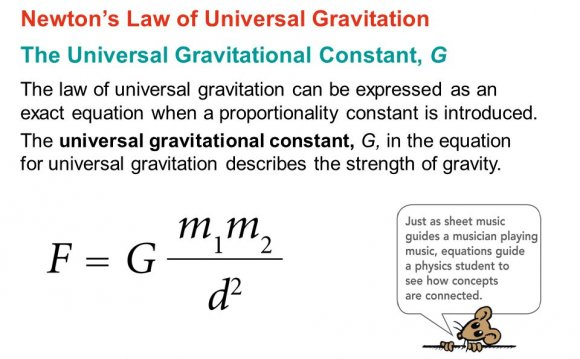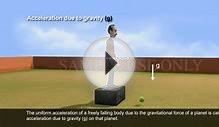# Universal gravitational constant equation

October 21, 2015The Gravitational Constant is a major element in the Universal Gravitation Equation, F = GMm/R2. The constant was not determined until many years after Isaac Newton first formulated his equation and was calculated as a result of what is called the Cavendish Experiment.

This experiment used a torsion balance device to attract lead balls together, measuring the torque on a wire and equating it to the gravitational force between the balls. Then by a complex derivation, the value of G was determined.

Questions you may have include:

• What is the background of the Cavendish Experiment?
• What is the experimental setup?
• What is the derived equation for G?

### Background of experiment

After Isaac Newton formulated the Universal Gravitation Equation in 1687, there really wasn't much interest in G. Most scientists simply considered it a proportionality constant. They were more interested in gravity than in gravitation.

In 1798, Henry Cavendish performed an experiment to determine the density of the Earth, which would be useful in astronomical measurements. He used a torsion balance invented by geologist John Mitchell to accurately measure the force of attraction between two masses. From this measurement, he determined the mass of the Earth and then its density. In Cavendish's published paper on the experiment, he gave the value for the density and mass of the Earth but never mentioned the value for G.

It wasn't until 1873 that other scientists repeated the experiment and documented the value for G. The value for G implied from Cavendish's experiment was very accurate and within 1% of present-day measurements.

Because his experiment ultimately determined the value for G, Cavendish has been often incorrectly given credit for determining the gravitational constant.

### Cavendish experiment setup

The Cavendish experiment uses a torsion balance to measure the weak gravitational force between lead balls. A torsion balance consists of a bar suspended at its middle by a thin wire or fiber. Twisting the wire requires a torque that is a function of the wire width and material.

The way it works is that the gravitational force attracting the balls together turns the bar, overcoming twisting resistance—or torque resistance—from the wire. That resistance is a function of angle turned and the torsion coefficient of the wire. At some angle, the torque resistance equals the gravitational force.

However, the inertia of the balls causes them to go slightly beyond the equilibrium point and thus create a harmonic oscillation around that point. The oscillation is also measured by the light reflected from the mirror. The rate of oscillation is then used to determine the spring constant or torsion coefficient of the wire, which is necessary in the final calculation of G.

It is truly a clever experiment.

Cavendish Experiment uses torque to measure Gravitation Constant

### Values and Dimensions

In Cavendish's original experiment, the following values were used:

Mass of large ball M = 158 kg (348 lbs)

Radius of large ball rM = 30.5 cm (12 in)

Mass of small ball M = 0.73 kg (1.6 lbs)

Radius of small ball rm = 5 cm (2 in)

Separation of large balls L = 1.86 m (73.3 in)

Separation of small balls L = 1.86 m (73.3 in)

Distance between large and small balls R = 0.225 m (8.85 in)

More recent experiments have used other values.

### Equation for G

The derived equation for G is:

G = 2π2LθRe2/T2M

where

• G is the Universal Gravitation Constant
• π is the Greek letter pi = 3.14...
• L is the length of the torsion bar
• is the angle the bar turns
• Re is the equilibrium point distance between M and M
• T is the oscillation frequency
• M is the mass of the larger object
Source: www.school-for-champions.com
##### INTERESTING VIDEOUniversal Gravitational Law - Solving problemsTHE GRAVITATION CONSTANT